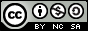- 目录 -
LeetCode 236 二叉树的最近公共祖先(递归)输入: root = [3,5,1,6,2,0,8,null,null,7,4], p = 5, q = 1



输入: root = [3,5,1,6,2,0,8,null,null,7,4], p = 5, q = 4



• 所有节点的值都是唯一的。
• p、q 为不同节点且均存在于给定的二叉树中。

## 思路

$$(f_{lson}&&f_{rson})||((x==p||x==q)&&({f_{lson}||f_{rson}})))$$

## 代码

  1 2 3 4 5 6 7 8 9 10 11 12 13 14 15 16 17 18 19 20 21 22 23 24 25 26 27 28 29  struct TreeNode { int val; TreeNode *left; TreeNode *right; TreeNode(int x) : val(x), left(NULL), right(NULL) {} }; class Solution { public: TreeNode *ans; // root的子树中是否包含p或者q bool dfs(TreeNode *root, TreeNode *p, TreeNode *q) { if (!root) return false; bool lson = dfs(root->left, p, q); bool rson = dfs(root->right, p, q); if ((lson && rson) || ((root->val == q->val || root->val == p->val) && (lson || rson))) ans = root; return lson || rson || root->val == p->val || root->val == q->val; } TreeNode *lowestCommonAncestor(TreeNode *root, TreeNode *p, TreeNode *q) { dfs(root, p, q); return ans; } };